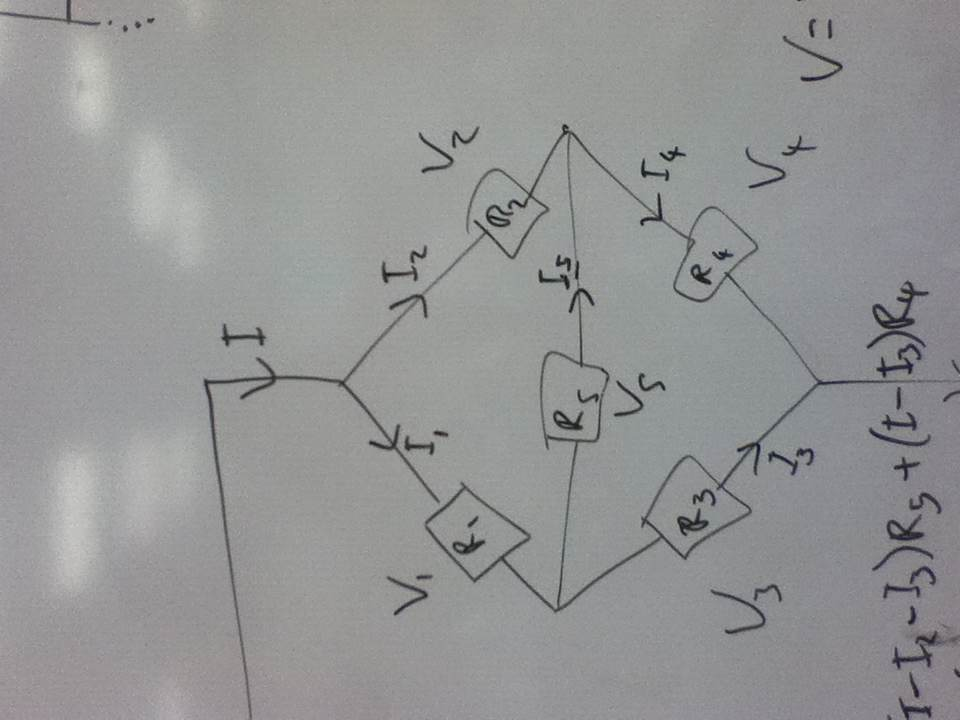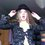# Non-trivial equivalent resistance.At school we have been learning the rules for equivalent resistance in series and parallel (which I covered at least two years ago, so I'm bored) and I asked my teacher about the circuit shown, which cannot be decomposed into a collection of series and parallel circuits.

I spent most of a lesson just bashing it with algebra, and in the end I found a three-line fraction for $R_{eq}$ in terms of $R_{1}$, $R_{2}$, $R_{3}$, $R_{4}$ and $R_{5}$.

So I was wondering: is there a nice method?Note by Sophie Crane
6 years, 9 months ago

This discussion board is a place to discuss our Daily Challenges and the math and science related to those challenges. Explanations are more than just a solution — they should explain the steps and thinking strategies that you used to obtain the solution. Comments should further the discussion of math and science.

When posting on Brilliant:

• Use the emojis to react to an explanation, whether you're congratulating a job well done , or just really confused .
• Ask specific questions about the challenge or the steps in somebody's explanation. Well-posed questions can add a lot to the discussion, but posting "I don't understand!" doesn't help anyone.
• Try to contribute something new to the discussion, whether it is an extension, generalization or other idea related to the challenge.

MarkdownAppears as
*italics* or _italics_ italics
**bold** or __bold__ bold
- bulleted- list
• bulleted
• list
1. numbered2. list
1. numbered
2. list
Note: you must add a full line of space before and after lists for them to show up correctly
paragraph 1paragraph 2

paragraph 1

paragraph 2

[example link](https://brilliant.org)example link
> This is a quote
This is a quote
    # I indented these lines
# 4 spaces, and now they show
# up as a code block.

print "hello world"
# I indented these lines
# 4 spaces, and now they show
# up as a code block.

print "hello world"
MathAppears as
Remember to wrap math in $$ ... $$ or $ ... $ to ensure proper formatting.
2 \times 3 $2 \times 3$
2^{34} $2^{34}$
a_{i-1} $a_{i-1}$
\frac{2}{3} $\frac{2}{3}$
\sqrt{2} $\sqrt{2}$
\sum_{i=1}^3 $\sum_{i=1}^3$
\sin \theta $\sin \theta$
\boxed{123} $\boxed{123}$

Sort by:

I think delta - star method can help .

- 6 years, 9 months ago

Can you give me an explanation/link to an explanation? Thanks

- 6 years, 8 months ago

@Sophie Crane It's trivial using Y-$\Delta$ transformation. Just apply this on any of the ends of $\text{R}_{5}$.

- 6 years, 8 months ago

Thank you very much. This is an excellent technique and I had never heard of it before. Awesome! :D

- 6 years, 8 months ago

If u use loop rule instead of junction rule, the expression would have been simpler. For making it even simpler u can assume the circuit to be connected across a good emf(I mean u can take different emfs if values of the resistances are known)

- 6 years, 9 months ago

I used both. The emf is an arbitrary value. All resistances are unknown, and are to be treated as algebraic variables. I am trying to find a general expression for the equivalent resistance in terms of these variables.

- 6 years, 9 months ago

That would be a very big formula

- 6 years, 9 months ago

It is. That's why I posted this.

- 6 years, 9 months ago

But u can still assume any value for emf as that wouldn't change the equivalent resistance.

- 6 years, 9 months ago

Correct me if I'm wrong, but isn't this the outline of a Wheatstone bridge? If $\dfrac{R_2}{R_4}=\dfrac{R_1}{R_3}$, then the equivalent can be easily calculated since the resistance $R_5$ will be ineffective. Is that condition given, or the variables $R_1,R_2,R_3,R_4,R_5$ can have any positive real value?

- 6 years, 7 months ago

You are correct on every count. The condition is that the resistors can take on any positive real value.

- 6 years, 7 months ago

I got a fraction of 8 terms over 4 terms

- 6 years, 7 months ago

Did you use delta-wye or something else?

- 6 years, 7 months ago